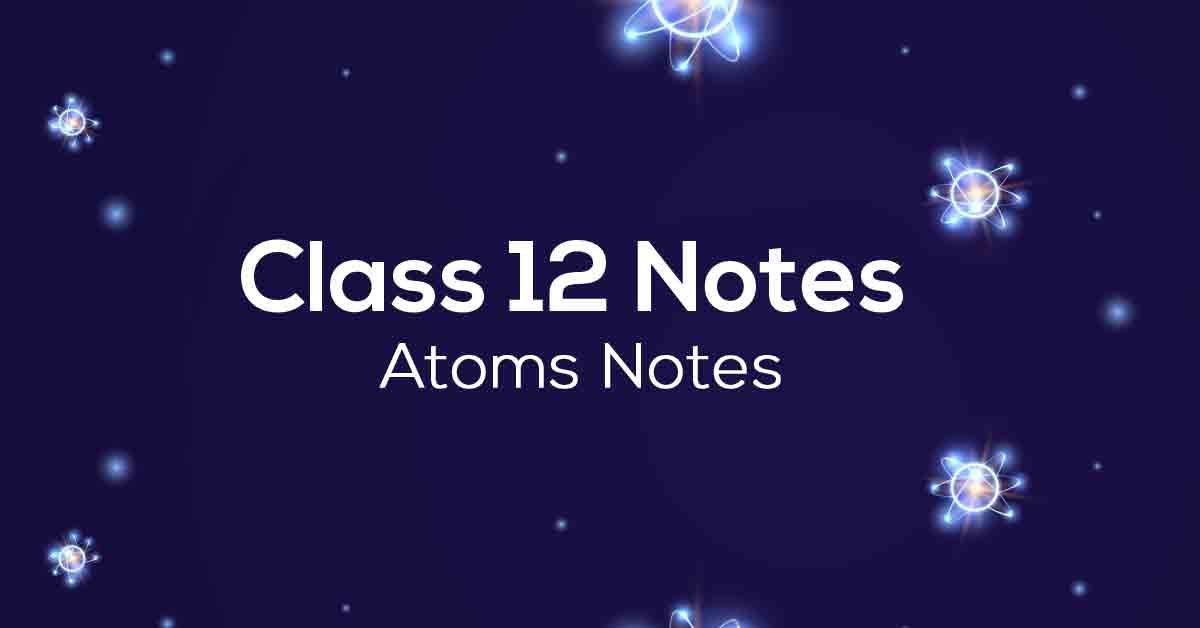Class 12th Physics Atoms NCERT Notes CBSE 2023

# Atoms Class 12 Notes# Chapter 12 Atoms

The twelfth chapter of Grade 12 Physics is Atoms. In this chapter, students will learn about concepts such as the atomic model, applications of X-rays, Mosley's law, and more. Students will deepen their understanding of various atomic concepts after consulting Vidyakul's NCERT notes prepared according to the latest program. understanding way.

Grade 12 Physics Chapter 12 NCERT notes contain answers to all questions in the text at the end of the chapter. Students taking the board exam should always practice NCERT questions for Atoms and related concepts. Scroll down to learn more about NCERT notes for Chapter 12 of Grade 12 Physics.

### Points to Remember

Below are some key takeaways from Class 12 Physics Chapter 12 Atoms:

• Atom is electrically neutral and thus contains an equal amount of both positive and negative charges.

• In Thomson’s model, the atom is a spherical cloud of positive charges with electrons embedded in it.

• As per Rutherford’s model, most of the mass of the atom and all its positive charge is concentrated in a small nucleus (typically one by ten thousand to the size of an atom), and the electrons revolve around it.

• Rutherford’s model says that atoms are unstable as the accelerated electrons revolving around the nucleus must spiral into the nucleus. This contradicts the stability of matter.

• Niel’s Bohr’s model on hydrogenic (single electron) atoms introduces three postulates and lays the foundations of quantum mechanics.

• de Broglie’s hypothesis that electrons have a wavelength λ=h/mvλ=h/mv explains Bohr’s quantized orbits by bringing in the wave-particle duality. The orbits correspond to the circular standing waves in which the circumference of the orbit equals a whole number of wavelengths.

### Topics and Sub-topics

In Chapter 12 of Grade 12 Physics, students explore atoms, their structure, the particles that makeup atoms, the properties and properties of nuclei, alpha particle trajectories, electrons, protons, and neutrons. The NCERT notes for Year 12 Physics Chapter 11 are based on the CBSE labeling scheme fromVidyakul.

After learning all of this chapter, students can practice Vidyakul questions and take a free trial test. The table below lists the important topics of this chapter.

:

 Sr. No. Topic Name Description 1 Introduction 2 Alpha-Particle Scattering And Rutherford’s Nuclear Model Of Atom 3 Alpha-Particle Trajectory 4 Electron Orbits 5 Atomic Spectra 6 Spectral Series 7 Bohr Model Of The Hydrogen Atom 8 Energy Levels 9 The Line Spectra Of The Hydrogen Atom 10 De Broglie’s Explanation Of Bohr’s Second Postulate Of Quantisation.

Download Vidyakul App for more Important videos, PDF's and Free video lectures.

### Few Important Questions

• What are ‘Alpha’ particles?

Alpha particles are composite particles consisting of two protons and two neutrons tightly bound together.

• What does the ‘Balmer series’ refer to?

The Balmer series is the name given to a series of spectral emission lines of the hydrogen atom that result from electron transitions from higher levels down to the energy level with principal quantum number 2.

• What is the ‘de Broglie hypothesis’?

De Broglie’s hypothesis of matter waves postulates that any particle of matter that has linear momentum is also a wave.

### Practice Questions

1. When the alpha-particle scattering experiment is repeated using a thin sheet of solid hydrogen in place of the gold foil. (Hydrogen is a solid at temperatures below 14K.) Find out the results.

2. Name the shortest wavelength present in the Paschen series of spectral lines?

3. When the atom makes a transition from the upper level to the lower level when the difference of 5 eV separates two energy levels in an atom, What is the frequency of radiation emitted?